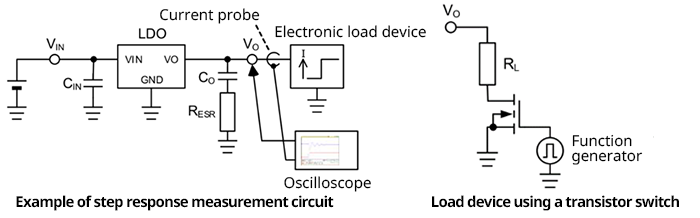### DC-DC｜Application

2021.07.28

・Some linear regulators may oscillate when an MLCC or other low-ESR capacitor is used as the output capacitor.

・In such cases, a resistor can be inserted in series with the output capacitor to increase the apparent ESR and create a phase lead so as to avoid oscillation.

・One general approach to deciding the value of the resistor to insert in series is to determine the phase margin of the linear regulator in the circuit.

・As one measure to use when the phase margin cannot be measured, the step response method can be employed to easily confirm the stability of the linear regulator.

Linear regulators developed in an era in which multilayer ceramic capacitors (hereafter MLCCs) were not widely used may cause a phase lag in the feedback loop, leading to oscillation when a low-ESR capacitor such as an MLCC is connected to the output. In this case, a resistor can be inserted in series with the output capacitor to increase the apparent ESR and cause a phase lead, thereby avoiding oscillation.

One straightforward approach to determining the value of the resistor to be inserted in series is to determine the actual phase margin of the linear regulator in the circuit using a frequency response analyzer (FRA). However, it may not be possible to use an FRA, or even if an FRA is available for use, a linear regulator with a fixed output voltage may have its feedback loop within an IC where it cannot be accessed, so that the FRA cannot be used in phase margin measurements. One possible measure in such cases is using what is called the step response method to confirm and optimize the stability of the linear regulator by simple means.

## About the Step Response Method

In the step response method, the output load (output current) is changed suddenly (stepped), and while using an oscilloscope to observe fluctuations in the output voltage (that is, the load transient response characteristic), adjustments are made to obtain a proper response. In this method, rigorous measurements of phase and gain are not possible, but even when using an FRA, the essence of the method is ultimately observation of the actual step response. In this sense, the method is empirically based, but can be considered to be an approach that depends on actual step responses.

Below is shown an example of a circuit for step response measurement. The setup is comparatively simple. An electronic load device is connected to the output of the linear regulator, and the load current is changed suddenly, monitoring the load current and output voltage on an oscilloscope.

When an electronic load device is not available, measurements can also be performed using a circuit that employs a transistor switch. A function generator is connected to the gate of an N-channel MOSFET and the transistor is turned on and off at high speed. When the transistor is off the current is 0 A, and when it is on a current equal to VO÷RL flows.The load current changes rapidly, for example from 0 A to the maximum output current of the linear regulator at a slew rate of 1 A/μs. Due to such sharp and large current steps, the output voltage essentially changes, stabilizing after a certain time has elapsed. The magnitude of this change and the time until convergence are optimized by adjusting the values of the relevant components (in this case, the value of RESR in the circuit diagram).